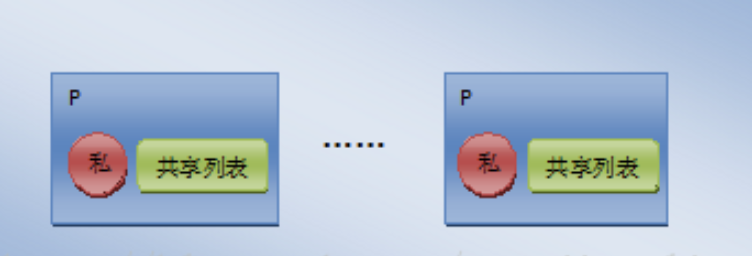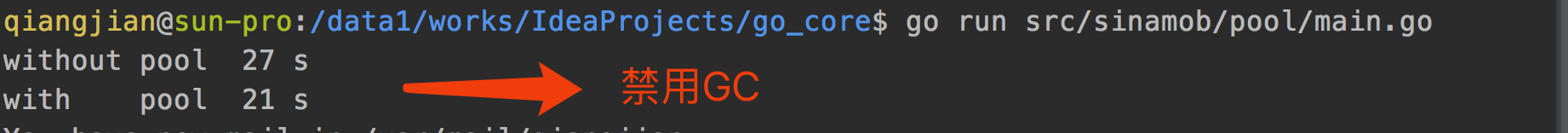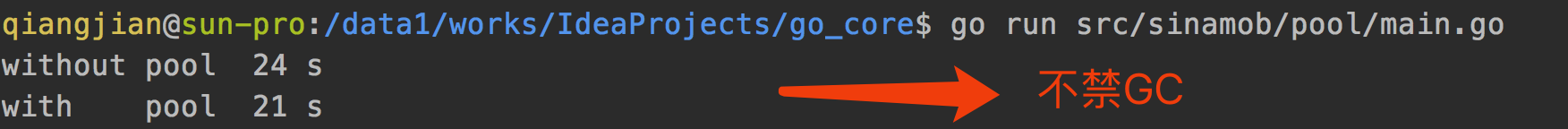# 深入Golang之sync.Pool详解

sync.Pool首先声明了两个结构体

// Local per-P Pool appendix.
type poolLocalInternal struct {
private interface{}   // Can be used only by the respective P.
shared  []interface{} // Can be used by any P.
Mutex                 // Protects shared.
}

type poolLocal struct {
poolLocalInternal

// Prevents false sharing on widespread platforms with
// 128 mod (cache line size) = 0 .
}


// Put adds x to the pool.
func (p *Pool) Put(x interface{}) {
if x == nil {
return
}
if race.Enabled {
if fastrand()%4 == 0 {
// Randomly drop x on floor.
return
}
race.Disable()
}
l := p.pin()
if l.private == nil {
l.private = x
x = nil
}
runtime_procUnpin()
if x != nil {
l.Lock()
l.shared = append(l.shared, x)
l.Unlock()
}
if race.Enabled {
race.Enable()
}
}


1. 如果放入的值为空，直接return.
2. 检查当前goroutine的是否设置对象池私有值，如果没有则将x赋值给其私有成员，并将x设置为nil。
3. 如果当前goroutine私有值已经被设置，那么将该值追加到共享列表。
func (p *Pool) Get() interface{} {
if race.Enabled {
race.Disable()
}
l := p.pin()
x := l.private
l.private = nil
runtime_procUnpin()
if x == nil {
l.Lock()
last := len(l.shared) - 1
if last >= 0 {
x = l.shared[last]
l.shared = l.shared[:last]
}
l.Unlock()
if x == nil {
x = p.getSlow()
}
}
if race.Enabled {
race.Enable()
if x != nil {
}
}
if x == nil && p.New != nil {
x = p.New()
}
return x
}

1. 尝试从本地P对应的那个本地池中获取一个对象值, 并从本地池冲删除该值。
2. 如果获取失败，那么从共享池中获取, 并从共享队列中删除该值。
3. 如果获取失败，那么从其他P的共享池中偷一个过来，并删除共享池中的该值(p.getSlow())。
4. 如果仍然失败，那么直接通过New()分配一个返回值，注意这个分配的值不会被放入池中。New()返回用户注册的New函数的值，如果用户未注册New，那么返回nil。func init() {
runtime_registerPoolCleanup(poolCleanup)
}


package main

import (
"sync"
"time"
"fmt"
)

var bytePool = sync.Pool{
New: func() interface{} {
b := make([]byte, 1024)
return &b
},
}

func main()  {
//defer
//debug.SetGCPercent(debug.SetGCPercent(-1))
a := time.Now().Unix()
for i:=0;i<1000000000;i++{
obj := make([]byte, 1024)
_ = obj
}
b := time.Now().Unix()

for j:=0;j<1000000000;j++  {
obj := bytePool.Get().(*[]byte)
_ = obj
bytePool.Put(obj)
}

c := time.Now().Unix()
fmt.Println("without pool ", b - a, "s")
fmt.Println("with    pool ", c - b, "s")
}## 总结：

posted @ 2018-09-26 12:27  sunsky303  阅读(19012)  评论(1编辑  收藏